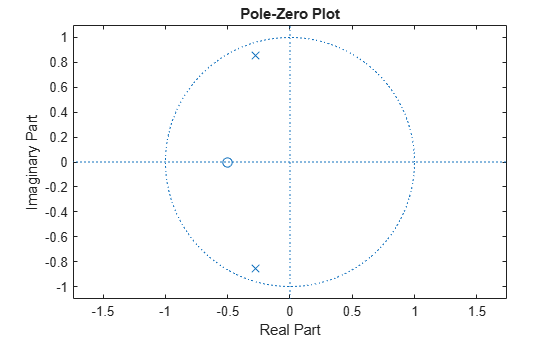# Zero-Pole Analysis

The `zplane` function plots poles and zeros of a linear system. For example, a simple filter with a zero at -1/2 and a complex pole pair at $0.9{e}^{-j2\pi 0.3}$ and $0.9{e}^{j2\pi 0.3}$ is

```zer = -0.5; pol = 0.9*exp(j*2*pi*[-0.3 0.3]');```

To view the pole-zero plot for this filter you can use `zplane`. Supply column vector arguments when the system is in pole-zero form.

`zplane(zer,pol)`For access to additional tools, use `fvtool`. First convert the poles and zeros to transfer function form, then call `fvtool`.

```[b,a] = zp2tf(zer,pol,1); fvtool(b,a)```Click the Pole/Zero Plot toolbar button, select Analysis > Pole/Zero Plot from the menu, or type the following code to see the plot.

`fvtool(b,a,'Analysis','polezero')`To use `zplane` for a system in transfer function form, supply row vector arguments. In this case, `zplane` finds the roots of the numerator and denominator using the `roots` function and plots the resulting zeros and poles.

`zplane(b,a)`See Discrete-Time System Models for details on zero-pole and transfer function representation of systems.